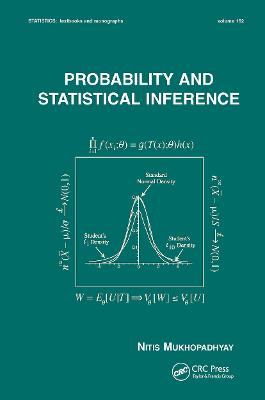•Probability and Statistical Inference - Statistics: A Series of Textbooks and Monographs 100 (Hardback)

(author)
£120.00
Hardback 665 Pages / Published: 22/03/2000
• We can order this from the publisher

Usually dispatched within 15 working days

Priced very competitively compared with other textbooks at this level!
This gracefully organized textbook reveals the rigorous theory of probability and statistical inference in the style of a tutorial, using worked examples, exercises, numerous figures and tables, and computer simulations to develop and illustrate concepts.

Beginning with an introduction to the basic ideas and techniques in probability theory and progressing to more rigorous topics, Probability and Statistical Inference
studies the Helmert transformation for normal distributions and the waiting time between failures for exponential distributions
develops notions of convergence in probability and distribution
spotlights the central limit theorem (CLT) for the sample variance
introduces sampling distributions and the Cornish-Fisher expansions
concentrates on the fundamentals of sufficiency, information, completeness, and ancillarity
explains Basu's Theorem as well as location, scale, and location-scale families of distributions
covers moment estimators, maximum likelihood estimators (MLE), Rao-Blackwellization, and the Cramer-Rao inequality
discusses uniformly minimum variance unbiased estimators (UMVUE) and Lehmann-Scheffe Theorems
focuses on the Neyman-Pearson theory of most powerful (MP) and uniformly most powerful (UMP) tests of hypotheses, as well as confidence intervals
includes the likelihood ratio (LR) tests for the mean, variance, and correlation coefficient
summarizes Bayesian methods
describes the monotone likelihood ratio (MLR) property
handles variance stabilizing transformations
provides a historical context for statistics and statistical discoveries
showcases great statisticians through biographical notes

Employing over 1400 equations to reinforce its subject matter, Probability and Statistical Inference is a groundbreaking text for first-year graduate and upper-level undergraduate courses in probability and statistical inference who have completed a calculus prerequisite, as well as a supplemental text for classes in Advanced Statistical Inference or Decision Theory.

Publisher: Taylor & Francis Inc
ISBN: 9780824703790
Number of pages: 665
Weight: 739 g
Dimensions: 229 x 152 x 37 mm